# One-Factor ANOVA (RM Design) Solution

You are interested in the effects of arousal on motor performance. A random sample of subjects perform a complex motor task under 3 conditions: no caffeine (low arousal), a small dose of caffeine (moderate arousal), and a large dose of caffeine (high arousal).
The dependent variable represents performance level: higher scores represent better performance.

This study resulted in the following data:

 Subject Low Difficulty Moderate Difficulty High Difficulty 1 15 17 19 2 2 7 4 3 11 12 6 4 13 15 5 5 12 12 7 6 2 18 11 7 8 12 5 8 9 14 2

Did the perceived level of caffeine significantly affect the participants’ performance (alpha = .05)? If your analysis reveals a significant overall effect, then make sure to explore all possible mean differences with a post-hoc analysis (same alpha).

The appropriate  test statistic for your study would be the value of the F
statistic that would result from  a One-Factor Repeated Measures Analysis of Variance (ANOVA):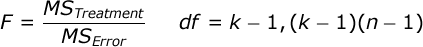The F statistic is equal to the ratio of the Mean Square
Treatment
divided by the Mean Square Error. k is
equal to the number of groups, and n is equal to the number of
scores in each treatment condition (n is also equal to the number of

Compute the test statistic. When we conduct an ANOVA, we typically
construct a Source Table
that details the sources of your variance and the corresponding degrees
of freedom:

 Source SS df MS F Treatment: Subjects: Error: Total:

To facilitate your ANOVA, you should compute the following statistics: a)  the sum of all scores (ΣX), the sum of all squared scores  (ΣX²), the overall number of scores (N), and the number of  scores in each treatment group (n). In our case, ΣX=238,  ΣX²=2964, N=24, and n=8. Compute these statistics for yourself  now.

Also, you should compute the sum of scores (T) for each treatment  condition:

 Low Arousal Moderate Arousal High Arousal T: 72 107 59

Now, you are ready to begin the Analysis of Variance. The Sum of Squares (SS) Total is equal to: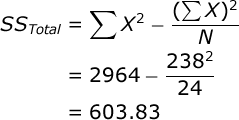SS for the treatment conditions is equal to: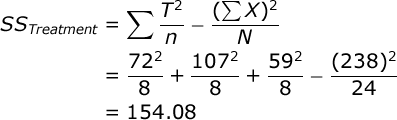Again, T is equal to the sum of scores in a particular treatment group.  Now, because this is a repeated-measures design, we need to compute the SS for  subjects. We will begin by computing the total (T) for each subject in the study:

 Subject Low Arousal Moderate Arousal High Arousal Total 1 15 17 19 51 2 2 7 4 13 3 11 12 6 29 4 13 15 5 33 5 12 12 7 31 6 2 18 11 31 7 8 12 5 25 8 9 14 2 25

Once we have a T score for each subject, you compute SS for subjects: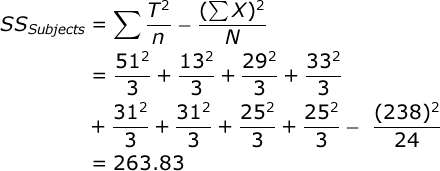Now, we can find SS Error by subtraction: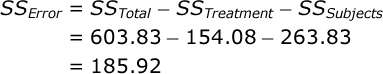Add these values to your Source Table. The df Total is equal to  the total number of scores minus one (N – 1). In our case this is: 24 – 1 = 23.  The df Treatment is equal to the number of treatment conditions  minus one (k – 1). In our case this is: 3 – 1 = 2.  The df Subjects is equal to the number of subjects minus one.  In our case this is: 8 – 1 = 7. The df Error is equal to the number  of treatment conditions minus one times the number of subjects minus one  (k – 1)(n – 1). In our case, this is: 2 * 7 = 14.

Each Mean Square (MS) is equal to the SS for that source divided by  the df for that source. In our case, MS  Treatment = 154.08 / 2 = 77.042,  and MS Error = 185.92 / 14 = 13.280.

Now you are ready to compute your test statistic. F is equal to: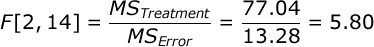Here is your completed Source Table:

 Source SS df MS F Treatment: 154.08 2 77.042 5.80 Subjects: 263.82 7 Error: 185.92 14 13.280 Total: 603.83 23

Conduct hypothesis test. In our study, the F statistic  will have 2 and 14 df, and alpha = .05.  If you look up the critical value of F in your  table of critical values, you will see that if your obtained  F is greater than 3.74, you would conclude that perceived  task difficulty significantly affected actual task performance  (i.e., that there exists one or more mean differences among the treatment  group means).

Since the obtained F (5.80) is greater than the critical value for  F (3.74), you would conclude that there was a significant  overall effect of perceived task difficulty on performance.

Compute effect size. We will compute the overall effect size two  ways. First let’s compute Overall Eta Squared, which is very simple: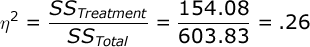Eta Squared is an estimate of the  proportion of variance in your  dependent variable that can be accounted for by variation in your independent  variable. However, the problem with  Eta Squared is that it will systematically over-estimate the variance  accounted for in your experiment; the smaller the sample size in your study,  the more biased Eta Squared will be.  So, we typically prefer to compute an unbiased estimate of the  variance accounted for.  Probably the best and most commonly used effect size statistic for a study like  ours would be Overall Omega Squared: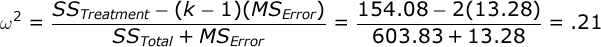So, we could conclude that our manipulation of caffeine level  accounted for about 21% of the variance in our participants’ performance.  However, computing effect size for a repeated-measures design is more  complicated than it is for the completely-randomized design.

Some would argue that in this design, subject variance is a systematic  source of variance that is not related to your hypothesis test.  They would suggest that we should disregard  subject variance and consider only treatment and error variance  in our analysis. Thus, it would be more appropriate to compute effect size  after we have removed subject variance from consideration.

If we follow this approach, we would compute Partial Eta Squared: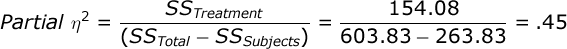…and Partial Omega Squared: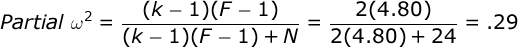Post-Hoc testing. The F statistic provides us with an  omnibus test of whether or not our independent variable affected our  dependent variable. It does not, however, help us to understand the exact  nature of this effect.

In our case, we know that there exists at least one significant mean  difference among our treatment groups, but we have no idea at this point  which particular mean difference might be significant. So, we should  follow up our ANOVA with a post-hoc test. We will examine all pair-wise comparisons among our treatment groups and figure out which  treatment group mean differences are statistically significant.

We will employ Tukey’s Honestly Significant Difference  (HSD) test. This will reveal to us what would constitute a  statistically significant mean difference between any two treatment group  means, controlling alpha over the course of all of these comparisons. In our study, an Honestly Significant Difference would be equal to: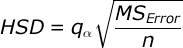where q is equal to the Studentized Range Statistic and is equal to the number of subjects. In our case, we have 8 subjects. When  alpha is equal to .05, you have 3 treatment groups, and you have 14 df  within-groups, when you examine your table  of critical values, you will find that  the critical value of the Studentized Range Statistic for this study is  equal to 3.701. So, the value  of a significant mean difference in this study would be equal to: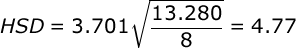Now, create a small table of the treatment group mean differences. Each  value in this table is equal to the mean of the row group minus  the mean of the column group:

 Treatment Group: 1 2 3 1. Low: -4.38 1.63 2. Mod Diff: 6.00 3. High Diff: Group Mean: 9.00 13.38 7.38

Then, compare the mean differences to your HSD. You will see that there is one significant pairwise comparison among  the treatment groups: the mean difference between the moderate-arousal and  the high-arousal conditions.